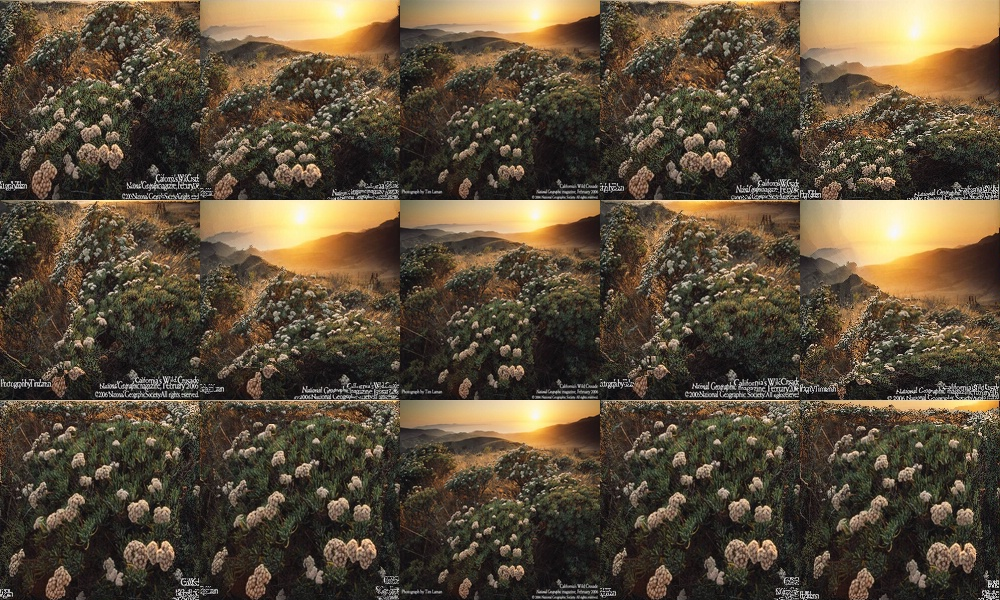ReTarget

Author V. C. Mohan

Of late seam carving tecnique is in news as an image resizing method that will carve out least interesting lines from image, thereby keeping interesting parts of image mostly unaffected.

Shai Avidan and Ariel Shamir in their paper titled 'improved seam carving for video retargetting' proposed some new criteria for application to video images. Incorporating this criteria( slightly modified) in addition to some more 'ReTarget' plugin is developed. This is similar to the seam carving plugin seamer in certain features, but differs a lot in its gamut and enables a better seam carving for videos.

It must be noted that the video retargeting is to enable a video image to be displayed on different sized display units ( cell phones, pdas etc;) losing least amount of the interesting detail of the original. For Example a face in the video may be more important than the background. However it must be kept in mind that the resulting image may have distorted perspective, if parameters are not chosen properly. It is also essential to note that the process time is proportional to a cubic power of input frame size and linear to number of seams to be carved. Retargeting 400X400 input to 200X200 size (excepting first frame) is not that slow. But if a 720X480 image is Retargetted to 200X200 it requires some patience.

Seam identification can be done by the energy parameter. 0 is backward energy, 1 forward energy, 2 total energy and 3 energy change

To ensure that the seams to be carved follow the seams of previous frame within limits, parameters are included. upto a total of 4 areas on image can be marked for either protection or deletion.If parts of areas of these masks overlap then the mask with higher number will prevail in that overlapped area. However due to various other overriding parameters(unless properly chosen),such markings may only be partly respected. These areas can translate linearly across from frame(field) to frame(field), but keep such translation not more than a few pixels per frame.

Limit usage to one scene or part of a scene with least camera panning or subject movement.

X and Y are the desired dimensions of the frame. Only reduction is possible

Input clip RGB format only and preferably be field based.

#Usage examples:-
im1 = lanczosresize(im1,400,400)# input RGB32 clip
# no protection or deletion
trm00 = ReTarget(im1,x =200,y =  200,energy = 0)
trm01 = ReTarget(im1,x =200,y =  200,energy = 1)
trm02 = ReTarget(im1,x =200,y =  200,energy = 2)
trm03 = ReTarget(im1,x =200,y =  200,energy = 3)
## delete a oval zone
trm0 = ReTarget(im1,x =200,y =  200,energy = 0,nm = 1, m1 = 1, m1x = 170, m1y = 300, m1w = 140, m1h = 200)
trm1 = ReTarget(im1,x = 200,y = 200, energy = 1, nm = 1, m1 = 1, m1x = 170, m1y = 300, m1w = 140, m1h = 200)
trm2 = ReTarget(im1,x =200,y =  200,energy = 2,nm = 1, m1 = 1, m1x = 170, m1y = 300, m1w = 140, m1h = 200)
trm3 = ReTarget(im1,x =200,y =  200,energy = 3,nm = 1, m1 = 1, m1x = 170, m1y = 300, m1w = 140, m1h = 200)
## protect a oval zone
trm10 = ReTarget(im1,x =200,y =  200,energy = 0,nm = 1, m1 = 0, m1x = 170, m1y = 300, m1w = 140, m1h = 200)
trm11 = ReTarget(im1,x = 200,y = 200, energy = 1, nm = 1, m1 = 0, m1x = 170, m1y = 300, m1w = 140, m1h = 200)
trm12 = ReTarget(im1,x =200,y =  200,energy = 2,nm = 1, m1 = 0, m1x = 170, m1y = 300, m1w = 140, m1h = 200)
trm13 = ReTarget(im1,x =200,y =  200,energy = 3,nm = 1, m1 = 0, m1x = 170, m1y = 300, m1w = 140, m1h = 200)
red = lanczosresize(im1,200,200)
s = stackhorizontal(trm00,trm01,red,trm02, trm03)
s1=stackhorizontal(trm0,trm1,red,trm2, trm3)
s2=stackhorizontal(trm10,trm11,red,trm12, trm13)
stackvertical(s,s1,s2)

Output below. Center panel in all rows is Resized image
All rows left is using Backward energy, on its right Forward energy, Rightmost is Changed energy and on its left total energy
Top row is without invoking protection or deletion
Middle row is with deletion of an oval zone
Bottom row is with protection of same oval zoneTo my index page down load plugin To Avisynth